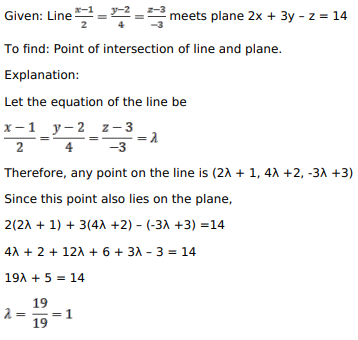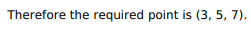# Mark against the correct answer in each of the following:

Question:

Mark against the correct answer in each of the following:

The line $\frac{x-1}{2}=\frac{y-2}{4}=\frac{z-3}{-3}$ meets the plane $2 x+3 y-z=14$ in the point

A. $(2,5,7)$

B. $(3,5,7)$

C. $(5,7,3)$

D. $(6,5,3)$

Solution: